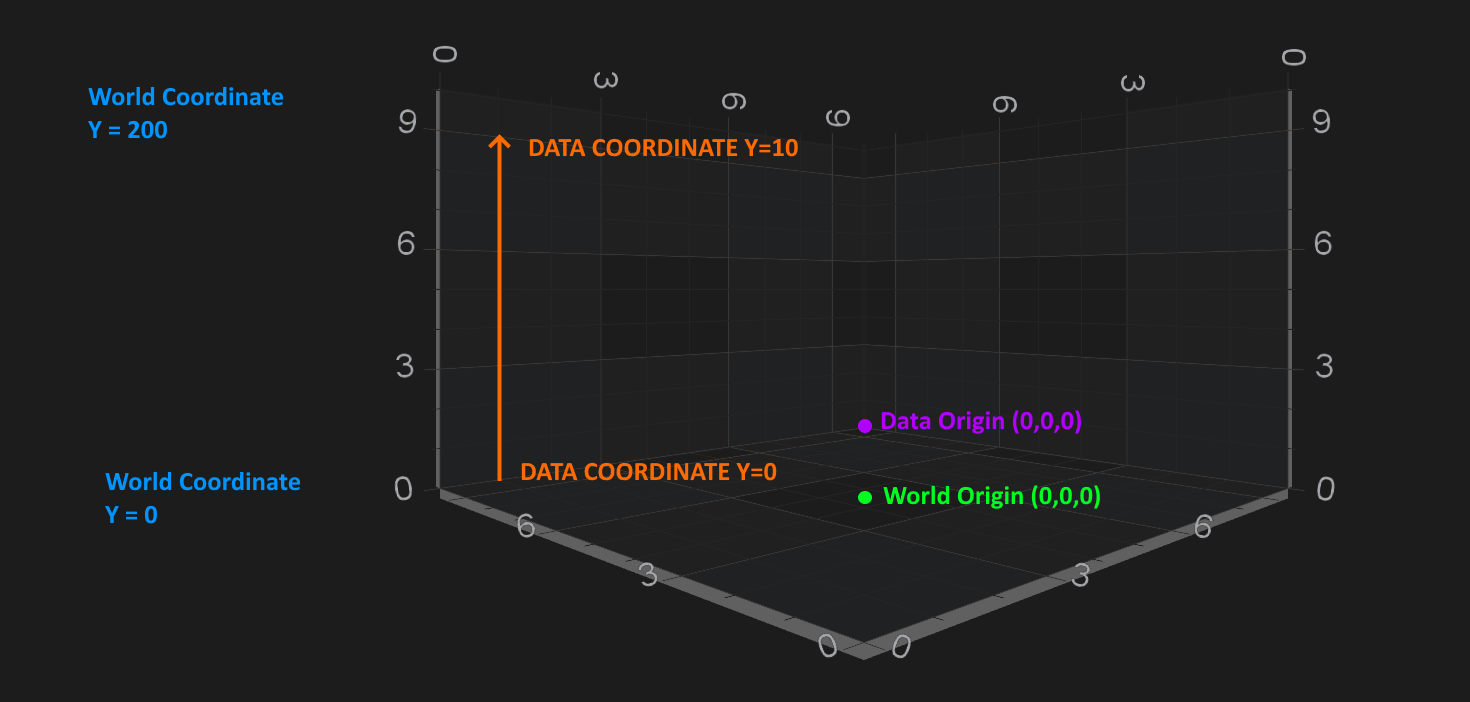iOS & macOS Charting Documentation - SciChart iOS & macOS Charts SDK v4.x

# Axis 3D APIs - Convert World to Data coordinates

SciChart iOS 3D provides a clean and simple API to transform 3D world coordinates to data-values and vice versa via the `ISCIAxisCore.currentCoordinateCalculator` API. The `ISCICoordinateCalculator` has the following methods suitable for conversions:

• `-[ISCICoordinateCalculator getCoordinateFrom:]` - expects a double representation of `data-value` and returns the corresponding pixel coordinate.
• `-[ISCICoordinateCalculator getDataValueFrom:]` - expects a `coordinate in pixels` and returns the closest data value to that coordinate (represented in double).

NOTE: For more information about `ISCICoordinateCalculator` - please read the Axis APIs - Convert Pixel to Data coordinates article.All Axes are responsible for converting between data-values and world-coordinates:

• `X-Axis` converts X data-values to X-World coordinates.
• `Y-Axis` converts Y data-values to Y-world coordinates.
• `Z-Axis` converts Z-data values to Z-world coordinates.

NOTE: For more information about the World Coordinates and Data Coordinates - please see the corresponding sections in the Coordinates in 3D Space article.

## Getting a CoordinateCalculator instance

There is a `ISCIAxisCore.currentCoordinateCalculator` property, which is `readonly`, and which provides a coordinate calculator instance which is only valid for the current render pass.

NOTE: If the `ISCIAxisCore.visibleRange` changes, the `ISCIChartSurface3D.worldDimensions` changes or the data changes - then the `ISCICoordinateCalculator` will be recreated under the hood, so it might give incorrect results. Hence, it’s advisable not to cache CoordinateCalculator instance, or cache it only for a short period of time, e.g. when using inside a loop.

## Converting between World to Data Coordinates

As mentioned above - data-values are converted to world coordinates via the `-[ISCICoordinateCalculator getCoordinateFrom:]` method. Also, Coordinates in pixels are converted back to chart data-values via the `-[ISCICoordinateCalculator getDataValueFrom:]` method.

Also, you might guess, converting World to Data-Coordinates and vice versa slightly differs for different axis types due to difference in underlying data-types. In particular the following ones:

Read on to get better understanding of such conversions.

### SCINumericAxis3D conversions

The simplest case is the `SCINumericAxis3D`. `ISCICoordinateCalculator` for NumericAxis 3D works the data-value as double. So let’s take our Scatter Chart 3D example, and try do some conversionsid<ISCICoordinateCalculator> calculator = yAxis.currentCoordinateCalculator; float coordinate = [calculator getCoordinateFrom:50]; // Convert back: double dataValue = [calculator getDataValueFrom:coordinate];
let calculator = yAxis.currentCoordinateCalculator! let coordinate = calculator.getCoordinate(50) // Convert back: let dataValue = calculator.getDataValue(coordinate)
var calculator = yAxis.CurrentCoordinateCalculator; var coordinate = calculator.GetCoordinate(50); // Convert back: var dataValue = calculator.GetDataValue(coordinate);

Y-Axis: VisibleRange = [-100, 100], Data-Value = 50, Pixel(World)-Coordinate = 149.25

NOTE: The exact data-values and coordinates might differ depending on your visibleRange, viewport etc…

### SCIDateAxis3D conversions

Similarly to `SCINumericAxis3D` - the `SCIDateAxis3D` is quite simple with one difference - it’s `ISCICoordinateCalculator` works with double representation of Date, which is timeIntervalSince1970. So let’s take our Date Axis 3D as an example, and try do some conversions.

NOTE: Since the `ISCICoordinateCalculator` works with double representation of Date in `timeIntervalSince1970`, you will need to do all the needed conversions on your own. See the code below:id<ISCICoordinateCalculator> calculator = zAxis.currentCoordinateCalculator; NSDate *date = [NSDate dateWithYear:2011 month:5 day:5]; float coordinate = [calculator getCoordinateFrom:date.timeIntervalSince1970]; // Convert back: double intervalSince1970 = [calculator getDataValueFrom:coordinate]; NSDate *dateValue = [NSDate dateWithTimeIntervalSince1970:intervalSince1970];
let calculator = zAxis.currentCoordinateCalculator! let date = NSDate(year: 2011, month: 5, day: 5)! let coordinate = calculator.getCoordinate(date.timeIntervalSince1970) // Convert back: let intervalSince1970 = calculator.getDataValue(coordinate) let dateValue = Date(timeIntervalSince1970: intervalSince1970)
var calculator = zAxis.CurrentCoordinateCalculator; var date = new DateTime(2011, 5, 5); var coordinate = calculator.GetCoordinate(date.ToUnixTime()); // Convert back: var intervalSince1970 = calculator.GetDataValue(coordinate); var dateValue = NSDate.FromTimeIntervalSince1970(intervalSince1970).FromDate();

Z-Axis: VisibleRange = [2019-05-01, 2019-05-08], Data-Value = “2019-05-05”, Pixel(World)-Coordinate = 170.857132

NOTE: The exact data-values and coordinates might differ depending on your visibleRange, viewport etc…